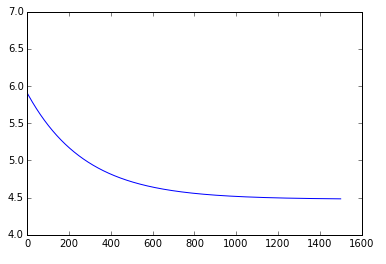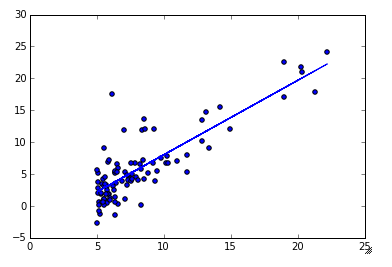I have previously done the Coursera Machine Learning exercises in Matlab. I thought, now that I am starting to get away from Matlab and use Python more, I should re-do the exercises in Python. This is exercise 1.

In :
```%matplotlib inline
import matplotlib.pyplot as plt
import numpy as np
import scipy
```

Part 1: Create an eye matrix. While this is incredibly simple, I want to make sure that I go through each step and provide a resulting document that a novice can follow long and understand what is happening.

In :
```A = np.identity(5)
print A
```

```[[ 1.  0.  0.  0.  0.]
[ 0.  1.  0.  0.  0.]
[ 0.  0.  1.  0.  0.]
[ 0.  0.  0.  1.  0.]
[ 0.  0.  0.  0.  1.]]
```

Part 2: Read in the data from ‘ex1data1.txt’ and plot it as a scatter plot

In :
```# Use the numpy genfromtext function to read the data
data = np.genfromtxt('/Users/matthewturner/Desktop/Coursera_machine_learning/'+
'machine-learning-ex1/ex1/ex1data1.txt', delimiter=',')
```
In :
```# Pull out the first column (theta0) into the variable X, and the 2nd column (theta1)
# into the variable y.  These variable names are consistent with the example.
X,y = data[:,0],data[:,1]

# Set m = the length of y (i.e., the number of observations).
m = len(y)
y = y.reshape(m,1)
```
In :
```plt.scatter(X,y)
```
Out:
`<matplotlib.collections.PathCollection at 0x1091f5d50>`In :
```# Add a column of ones to the data
X = np.c_[np.ones((m,1)),X]
theta = np.zeros((2,1))

iterations = 1500
alpha = 0.01
```
In :
```def costFuncIter(X,y,theta):
cum_sum = 0
for i in range(m):
h = np.dot(X[i],theta) - y[i]
cum_sum += 1.0/(2*m) * h * h
return cum_sum

J = costFuncIter(X,y,theta)
print J
```
```[ 32.07273388]
```
In :
```# Compute and display the initial cost function
def costFunc(X,y,theta):
h = np.dot(X,theta)-y
h2 = np.dot(h.T,h)
J = 1./(2*m) * h2
return J[0,0]

J = costFunc(X,y,theta)
print J
```
```32.0727338775
```
In :
```# Run the Gradient Descent Algorithm
J_history = np.zeros(iterations)

for iter in range(iterations):
h = np.dot(X,theta) - y
h_X = np.dot(X.T,h)

temp = theta

temp -= alpha / m * h_X

theta = temp

J_history[iter] = costFunc(X,y,theta)

return theta,J_history

print theta
```
```[[-3.63029144]
[ 1.16636235]]
```
In :
```# Plot the timeseries of cost function
plt.plot(J_history)
```
Out:
`[<matplotlib.lines.Line2D at 0x109fcfd90>]`In :
```# Plot the observations and the best fit line that we just solved for
plt.scatter(X[:,1],y)
plt.plot(X[:,1],np.dot(X,theta))
```
Out:
`[<matplotlib.lines.Line2D at 0x10a108810>]`In :
```# Make the 2 predictions
predict1 = np.array([1,3.5]).dot(theta)
predict2 = np.array([1,7]).dot(theta)
print predict1
print predict2
```
```[ 0.45197679]
[ 4.53424501]
```

Part 3: Now we do the multi variable exercise

In :
```# Use the numpy genfromtext function to read the data
data2 = np.genfromtxt('/Users/matthewturner/Desktop/Coursera_machine_learning/'+
'machine-learning-ex1/ex1/ex1data2.txt', delimiter=',')
```
In :
```# Pull out the first column (theta0) into the variable X, and the 2nd column (theta1)
# into the variable y.  These variable names are consistent with the example.
X2,y2 = data2[:,0:2],data2[:,2]

# Set m = the length of y (i.e., the number of observations).
m2 = len(y2)
y2 = y2.reshape(m2,1)
```

Part 3.1: Feature Normalization

In :
```def featureNormalize(X):
mu = np.mean(X,axis=0)
sigma = np.std(X,axis=0)
X_norm = ( X - mu ) / sigma
return X_norm, mu, sigma

X_norm, mu, sigma = featureNormalize(X2)
```
In :
```# Add the column of ones to X2
X2 = np.c_[np.ones((m2,1)),X_norm]
```

In :
```def gradientDescentMulti(X2,y2,theta2,alpha2,iterations2):
J_history2 = np.zeros(iterations2)

max1 = np.shape(X2)
alpha_over_m = alpha2 / m2
for iter in range(iterations2):
temp2 = theta2
h2 = np.dot(X2,temp2.reshape(max1,1))-y2
h3 = np.dot(X2.T,h2)
temp2 = temp2.reshape(max1,1) - alpha_over_m * h3
theta2 = temp2

J_history2[iter] = costFunc(X2,y2,theta2)

return theta2,J_history2

theta2 = np.zeros(np.shape(X2))
alpha2 = 0.1
iterations2 = 400

print theta2
```
```[[ 340412.65957447]
[ 109447.79558639]
[  -6578.3539709 ]]
```
In :
```plt.plot(J_history2)
```
Out:
`[<matplotlib.lines.Line2D at 0x10a591fd0>]`Part 3.3: Normal Equation

In :
```def normalEqn(X,y):
pinv_X = np.linalg.pinv(np.dot(X.T,X))
X_y = np.dot(X.T,y)
theta = np.dot(pinv_X,X_y)
return np.linalg.inv(X.T.dot( X )).dot( X.T ).dot( y )

theta3 = normalEqn(X2,y2)
print theta3
```
```[[ 340412.65957447]
[ 109447.79646964]
[  -6578.35485416]]
```
In :
```# Predict proce of 1650 sq foot house with 3 bedrooms
x_predict = [1650,3]
normalized_predict = (x_predict - mu)/sigma
normalized_predict = np.insert(normalized_predict,0,1)
predict3 = np.dot(normalized_predict,theta2)

print predict3
```
```[ 293081.46452917]
```

## One thought on “Coursera Machine Learning In Python (Exercise 1)”

1.I really don't know how to express my thanks to you.

This site uses Akismet to reduce spam. Learn how your comment data is processed.